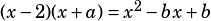Hey Mike,
A math question for the new PSATs,
If x-2 is a factor of x^2 – bx + b, where b is a constant, what is the value of b?

Thanks,
Brian.

From what you’re given, you know that, for some constant a. From that, you know that –2 + a–b, and that –2a = b.

Multiply that second equation by –1, and then you can do some solvin’.

–2 + a = –b
2a = –b

–2 + a = 2a
–2 = a

Confirm that works by FOILing:Yep, that works!

So that’s the algebraic solution. I suspect that once you’ve seen a few questions like this, you might be able to see it without doing the algebra.